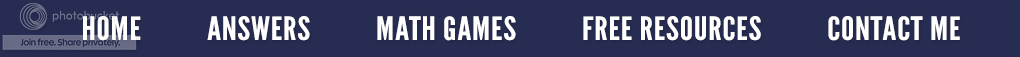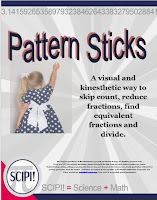The Beauty of Math Patterns

Some people say mathematics is the science of patterns which I think is a pretty accurate description. Not only do patterns take on many forms, but they occur in every part of mathematics. But then again patterns occur in other disciplines as well. They can be sequential, spatial, temporal, and even linguistic.

Recognizing number patterns is an important problem-solving skill. If you recognize a pattern when looking systematically at specific examples, that pattern can then be used to make things easier when needing a solution to a problem.

Mathematics is especially useful when it helps you to predict or make educated guesses, thus we are able to make many common assumptions based on reoccurring patterns. Let’s look at our first pattern below to see what we can discover.

What can you say about the multiplicand? (the number that is or is to be multiplied by another. In the problem 8 × 32, the multiplicand is 32.) Did you notice it is multiples of 9? What number is missing in the multiplier?

Now look at the product or answer. That’s an easy pattern to see! Use a calculator to find out what would happen if you multiplied 12,345,679 by 90, by 99 or by 108? Does another pattern develop or does the pattern end?

Here is a similar pattern that uses the multiples of 9. How is the multiplier in this pattern different from the ones in the problems above? Look at the first digit of each answer (it is highlighted). Notice how it increases by 1 each time. Now, observe the last digit of each answer. What pattern do you see there? Using a calculator, determine if the pattern continues or ends.
Recognizing, deciphering and understanding patterns are essential for several reasons. First, it aids in the development of problem solving skills. Secondly, patterns provide a clear understanding of mathematical relationships. Next, the knowledge of patterns is very helpful when transferred into other fields of study such as science or predicting the weather. But more importantly, understanding patterns provides the basis for comprehending Algebra since a major component of solving algebraic problems\$3.00

is data analysis which, in turn, is related to the understanding of patterns. Without being able to recognize the development of patterns, the ability to be proficient in Algebra will be limited.

So everywhere you go today, look for patterns. Then think about how that pattern is related to mathematics. Better yet, share the pattern you see by making a comment on this blog posting.

---------------------------------------------------------------------------
Check out the resource Pattern Sticks. It might be something you will want to use in your classroom.